## Introduction to Functions and Relations

• A mathematical relation is a set of ordered pairs whose set of first components is called the domain while the set of second components is called the range.
• A relation is a rule that relates values of the first set of values (called the domain) to the second set of values (called the range).
• A function is a relation in which each element in the domain corresponds to one and only one element in the range.
• All functions are relations but not all relations are defined as functions.
• Assume that M and N are sets. A function $f$ from M to N, signified by $f:M\to N$, is a set of ordered pairs  wherein  and every element of M is paired exactly one element of N.
• A function is typically denoted by a symbol . The notation $y=f\left(x\right)$ states that y element is the value of .
• The notation $f\left(x\right)$ is read as "".
• The domain is the set of all permissible values of $x$ where $x$ denotes the independent variable.
• The range is the set of all resulting values of $y$, where $y$ denotes the dependent variable.

## Representations of Functions and Examples

The following are typical ways to represent functions: set of ordered pairs, table of values, mapping diagram, graphs, and equation.

• Using Set of Ordered Pairs

Example 1. Let . Relation A represents a function because no two ordered pairs have the same first element. It is also a function from X to Y. The domain is X and the image of this function is Y.

• Using Table of Values

Example 2. Study the table of values below.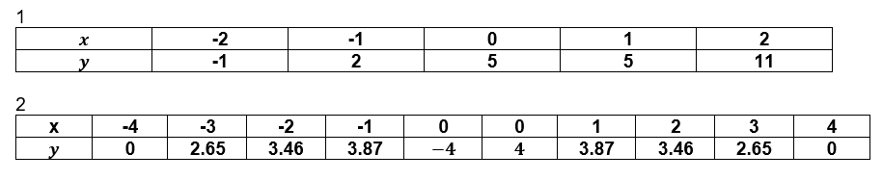Table 1 describes a function since no two ordered pairs have the same first element. On the other hand, table 2 does not represent a function since it has .

• Using Mapping Diagram

Study the different mapping diagrams below.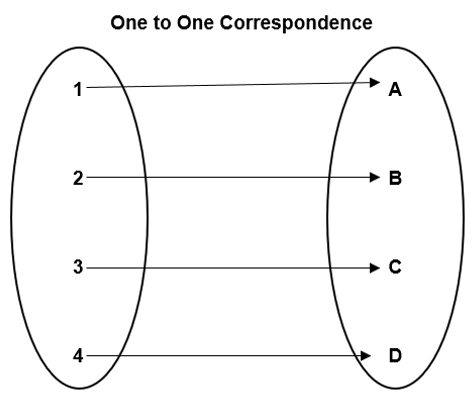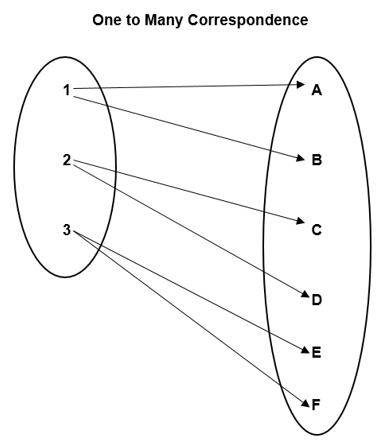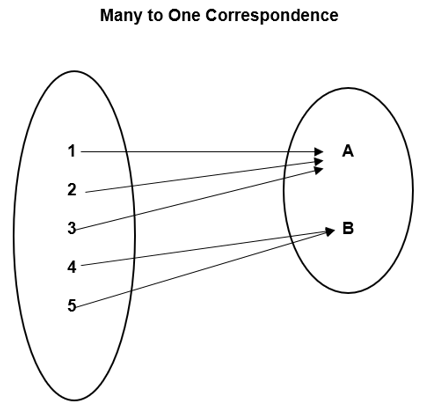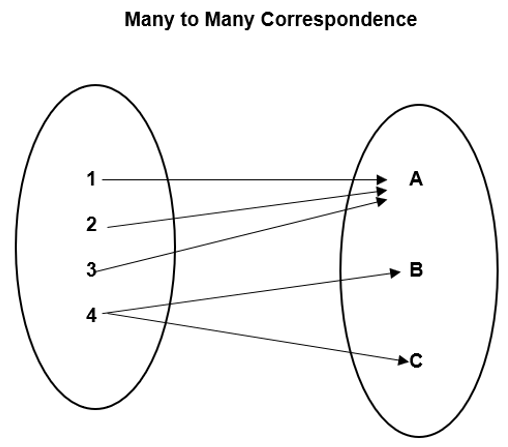One-to-One correspondence and Many-to-One Correspondence represent functions, while One-to-Many Correspondence and Many-to-Many Correspondence do not define functions.

• Function as a Graph

How do we determine if the graph of a relation defines a function? In this case, use the Vertical Line Test. By sketching a vertical line, it must intersect the graph at most once. Study the graphs below:

1.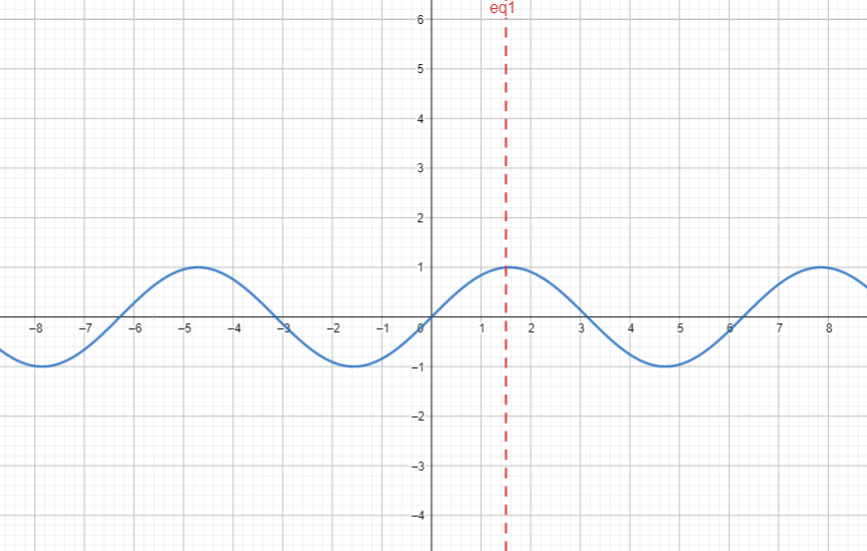2.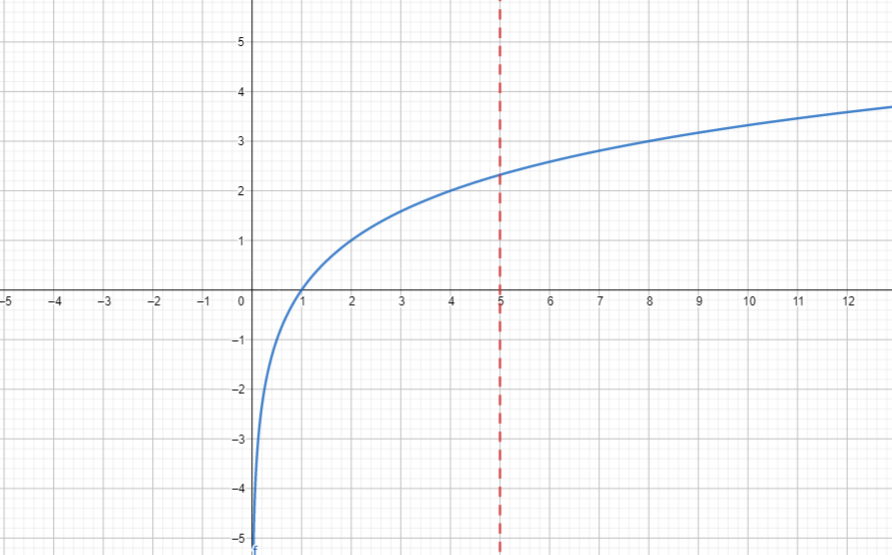3.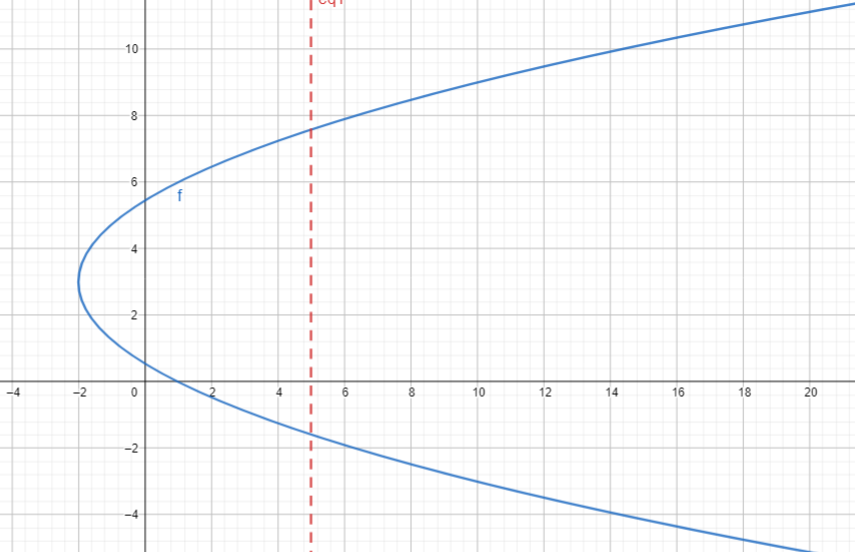Graph 1 and Graph 2 represent a function, while Graph 3 does not define a function.

• Function as an Equation
• Functions can be represented in different forms mathematically.
• If y is nonlinear given that its degree is even, the given function is not a function.
• Equation of function should determine y as a function of x. Recall that in a function, the concept of dependence is important.
• The variable y is the dependent variable while the variable x is the independent variable.

## Basic Types of Functions

In general, functions are classified into two types - Algebraic and Transcendental.

Functions are basically categorized in terms of the family of curves. The parent function is used to represent the simplest form among the family of functions. In doing transformations, the graphs of parent functions are served as bases.

Here are some basic types of functions:

• Polynomial Functions:
• Linear Functions
• Cubic Functions
• Quartic Functions
• Quintic Functions, etc.
• Absolute Value Functions
• Square Root Functions
• Greatest-Integer Functions (or Floor Functions)
• Ceiling Functions
• Piecewise-Defined Functions
• Trigonometric Functions and Inverse Trigonometric Functions
• Exponential and Logarithmic Functions

A function is even if $f\left(-x\right)=f\left(x\right)$ for all x in the domain of $f$, while a function is odd if $f\left(-x\right)=-f\left(x\right)$ for all x in the domain of $f$. Otherwise, a function is neither odd or even if it fails to achieve the algebraic substitution of .

## Cheat Sheet

• The equation ${y}^{5}-3{x}^{2}=27$ defines a function.
• On the other hand, the equation ${y}^{4}-{x}^{2}+5x-7y=6$ does not define a function.
• The equation $\frac{{x}^{2}-3}{x}=\left|3y-1\right|$ does not represent a function.
• The equation $x=\frac{x-1}{{x}^{2}-1}$ is not a representation of function since it only involves an independent variable $x$.
• The equation $x=9$ is not a function because it has a single value of $x$ that can generate different values of y.
• The equation $y=\frac{\left|5x-3\right|}{x+1}$ represents a function.

## Blunder Areas

• All equations can show a mathematical relation but not all of them can represent a function.
• The relation $y=±\sqrt{{x}^{2}-3}$ becomes a function if we treat this as .
• An empty set $\left\{\right\}$ is a function.